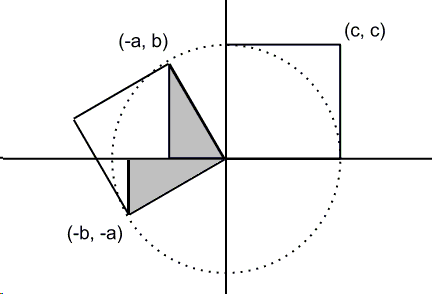# Pythagorean Theorem via the Area Determinant

This is a well known fact in linear algebra that a $2\times 2$ determinant $\left| \begin{array}{cc} a & b \\ c & d \end{array} \right| = ad - bc$ defines the signed area of the parallelogram on two vectors $\left( \begin{array}{c} a \\ c \end{array} \right)$ and $\left( \begin{array}{c} b \\ d \end{array}\right)$.

John Molokach applied that property of determinants to the following diagram:There are two squares with side $c$ and area $c^{2}$. One of them is identified as a parallelogram on vectors $\left( \begin{array}{c} -a \\ b \end{array} \right)$ and $\left( \begin{array}{c} -b \\ -a \end{array} \right)$. The area of the parallelogram is expressed as $|(-a)(-a)-b(-b)|=a^{2}+b^{2}$. Since the two areas are equal, $c^{2}=a^{2}+b^{2}$, the Pythagorean theorem.

John accompanied his diagram by a telling formula:

$\Bigg|\left| \begin{array}{cc} c & 0 \\ 0 & c \end{array} \right|\Bigg| = \Bigg|\left| \begin{array}{cc} -a & b \\ -b & -a \end{array} \right|\Bigg|$